##### Circuit Analysis For Dummies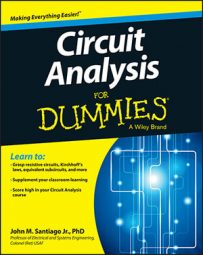A Thévenin or Norton equivalent circuit is valuable for analyzing the source and load parts of a circuit. Thévenin’s and Norton’s theorems allow you to replace a complicated array of independent sources and resistors, turning the source circuit into a single independent source connected with a single resistor.

You commonly use the Thévenin equivalent when you want circuit devices to be connected in series and the Norton equivalent when you want devices to be connected in parallel with the load. You can then use the voltage divider technique for a series circuit to obtain the load voltage, or you can use the current divider technique for a parallel circuit to obtain the load current.

## Apply source transformation to circuit analysis

To transform a circuit using the Thévenin or Norton approach, you need to know both the Thévenin voltage (open-circuit voltage) and the Norton current (short-circuit current). But you don’t need to find the Thévenin and Norton equivalent circuits separately.

After you figure out the Thévenin equivalent for a circuit, you can find the Norton equivalent using source transformation. Or if you figure out the Norton equivalent first, source transformation lets you find the Thévenin equivalent.

For example, if you already have the Thévenin equivalent circuit, then obtaining the Norton equivalent is a piece of cake. You perform the source transformation to convert the Thévenin voltage source connected in series with the Thévenin resistance into a current source connected in parallel with the Thévenin resistance.

The result is the Norton equivalent: The current source is the Norton current source, and the Thévenin resistance is the Norton resistance.

## Find the Thévenin equivalent of a circuit with multiple independent sources

You can use the Thévenin approach for circuits that have multiple independent sources. In some cases, you can use source transformation techniques to find the Thévenin resistor RT without actually computing voc and isc.

For example, consider Circuit A shown here. In this circuit, the voltage source vs and resistors R1 and R2 are connected in series.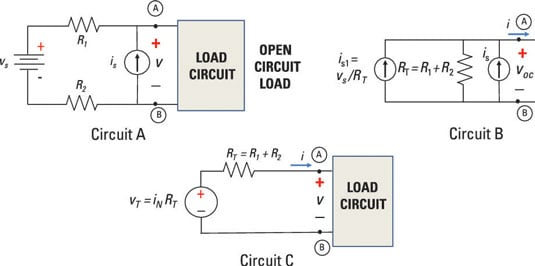When you remove independent sources vS1 and is in Circuit A, this series combination of resistors produces the following total Thévenin resistance: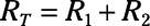You can then use source transformation to convert the Thévenin voltage source, which is connected in series to Thévenin resistance RT, into a current source that’s connected in parallel with RT. Here’s your current source: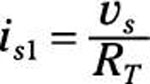Circuit B shows the transformed circuit with two independent current sources.

Because independent current sources are in parallel and point in the same direction, you can add up the two source currents, which produces the equivalent Norton current, iN: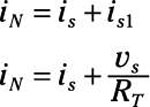Circuit B shows the combination of the two current sources. When you combine the two current sources into one single current source connected in parallel with one resistor, you have the Norton equivalent.

You can convert the current source iN in parallel with RT to a voltage source in series with RT using the following source transformation equation: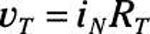Circuit C is the Thévenin equivalent consisting of one voltage source connected in series with a single equivalent resistor, RT.